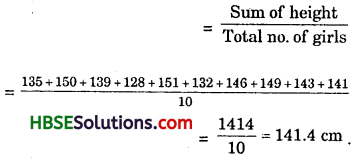# HBSE 7th Class Maths Solutions Chapter 3 Data Handling Ex 3.1

Haryana State Board HBSE 7th Class Maths Solutions Chapter 3 Data Handling Ex 3.1 Textbook Exercise Questions and Answers.

## Haryana Board 7th Class Maths Solutions Chapter 3 Data Handling Exercise 3.1

Question 1.
Find the range of heights of 10 students of your class.
Solution:
The height (in feet) of 10 students of my class VII,
= 3.5, 4, 5, 4, 3, 5, 4, 3.5, 4, 6.
∴ Range of the heights (in feet) of the students = 6-3 = 3 Feet.

Question 2.
Organise the following marks in a class assessment, in a tabular form.
(i) Which number is the highest ?
(ii) Which number is the lowest ?
(iii) What is the range of the data ?
(iv) Find the arithmetic mean.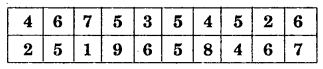Solution:
Let us put the data in a tabular form :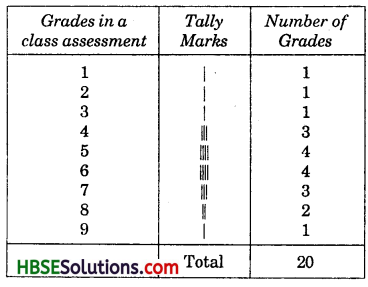(i) 9 is the highest grade.
(ii) 1 is the lowest grade.
(wi) Range of the data = 9-1 = 8
(iv) Arithmetic Mean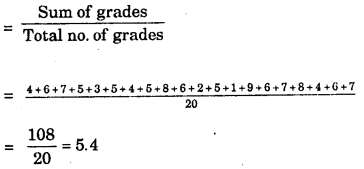Question 3.
Find the mean of first five whole numbers.
Solution:
Mean of first 5 whole number
$$\frac{0+1+2+3+4}{5}=\frac{10}{5}=2$$

Question 4.
A cricketer scores the following runs in eight innings: 58, 76,40,35,48,45, 0,100. Find the mean score.
Solution:
Mean score = $$\frac{\text { Sum of scores }}{\text { Total no. of innings }}$$
= $$\frac{58+76+40+35+48+45+0+100}{8}=\frac{402}{8}=$$ = 50.25

Question 5.
Following table shows the points of scores each player scored in four games: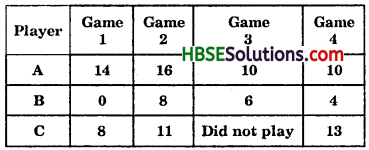(i) Find the mean to determine A’s average number of points scored per game.
(ii) To find the mean number of points per game for C, would you divide the total points by 3 or by 4 1 Why ?
(Hi) B played in all the four games. How would you find the mean ?
(iv) Who is the best performer ?
Solution:
(i) Mean of A
$$\frac{14+16+10+10}{4}=\frac{50}{4}=\frac{25}{2}$$ = 12.5

(ii) Mean of C = $$\frac{8+11+13}{3}=\frac{32}{3}$$ = 10.66
because three players play.
(iii) Mean of B
= $$\frac{0+8+6+4}{4}=\frac{18}{4}=\frac{9}{2}$$ = 4.5

(iv) Mean of A > Mean of C > Mean of B.
∴ A is the best performer.Question 6.
The marks (out of 100) obtained by a group of students in a science test are 85, 76, 90, 85, 39, 48, 56, 95, 81 and 75. Find the :
(i) Highest and the lowest mark obtained by the students.
(ii) Range of the marks obtained.
(iii) Mean marks obtained by the group.
Solution:
(i) Highest marks of the students = 95
Lowest marks of the students = 39 (ii) Range of the marks = highest marks – lowest marks = 95 – 39 = 56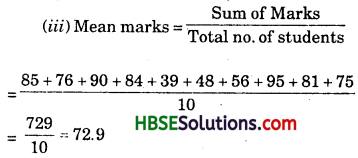Question 7.
The enrolment of a school during six consecutive years was as follows: 1555, 1670,1750,2013,2540,2820.
Find the mean enrolment of the school for this period.
Solution: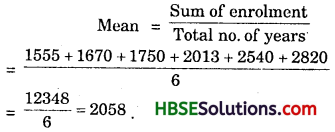Question 7.
The rainfall (in mm) in a city on 7 days of a certain week was recorded as follows :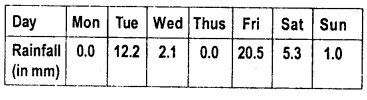(i) Find the range of the rainfall in the above data.
(ii) Find the mean rainfall for the week.
(iii) On how many days was the rainfall less
than the mean rainfall. HBSESolutions.com
Solution:
(i) Range of rainfall
= highest rainfall – lowest rainfall.
= 20.5 – 0.0 = 20.5 mm

(ii) Mean rainfall = $$\frac{\text { Sum of rainfall }}{\text { Total no. of days }}$$
= $$\frac{0.0+12.2+2.1+0.0+20.5+5.3+1.0}{7}$$
= $$\frac{41.1}{7}$$ = 5.87 mm

(iii) 5 days (Mon, Wed, Thus, Sat, Sun).Question 8.
The heights of 10 girls were measured in cm and the results are as follows: 135,150,139,128,151,132,146,149, 143,141.
(i) What is the height of the tallest girl ?
(ii) What is the height of the shortest girl ?
(iii) What is the range of the data ?
(iv) What is the mean of height the girls ?
(v) How many girls have heights more than the mean height.
Solution:
(i) 151 cm. is the height of the tallest girl.
(ii) 128 cm. is the height of the shortest girl.
(iii) Range of data = highest height – shortest height = 151 – 128 = 23 cm. ;
(iv) Mean height of the girls